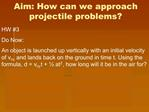DownloadDownload PresentationAim: How can we approach projectile problems

# Aim: How can we approach projectile problems

Download Presentation## Aim: How can we approach projectile problems

- - - - - - - - - - - - - - - - - - - - - - - - - - - E N D - - - - - - - - - - - - - - - - - - - - - - - - - - -
##### Presentation Transcript

1. Aim: How can we approach projectile problems?

2. Separate Components Velocity, acceleration, and distance have both horizontal (x) component, and vertical (y) component

3. Horizontally Fired Objects Horizontal velocity is constant:

4. Vertical Components For the vertical motion, the object is in free fall:

5. Projectiles Fired at an Angle Unlike horizontal projectiles, there are both vox and voy Initial velocity can be resolved into x and y components

6. Now what? Since you can determine your vox and voy, the rest we can figure out!!! Horizontal velocity is still constant Horizontal acceleration is still zero Vertical acceleration is -10 m/s2 (just like in vertically fired objects) Vertical vf at max height is 0 m/s (just like in vertically fired objects) Vertical displacement is 0 m (the object starts on the ground and ends on the ground) Time to max height = � total time

7. Time Vertical and Horizontal time are equal: